# Maths - Monads and Algebras

Given a monad: T: C -> C , 1 =>η T <=µ T denoted by the triple <T,η,µ>

An algebra for a monad <T,η,µ> is an object AC equipped with an 'action': a morphism θ:TA->A so that the following diagrams commute:

 Axioms of an algebra A: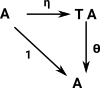### Relationship to an Algebraic Theory

If the monad T represents an algebraic theory.
Then an 'algebra for a monad' represents a model for the algebraic theory, that is, an implementation of it.

 Monad an algebraic theory Syntax Holds all the operations that may be needed. Let us form algebras Algebra for a monad a model for the algebraic theory Semantics An implementation of those operations. Let us evaluate (collapse) algebras

### Informal Introduction

 We can think of the endofunctor of a monad in terms of an expression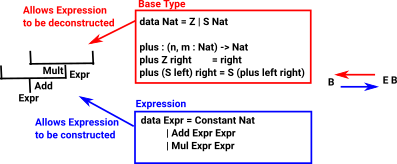We are first introduced to 'algebra' as a part of mathematics where we can use letters (variables) to stand in for numbers that are unknown or we don't wish to use the literal value. We can then use this to express axioms like: x+y =y+x.

Here we relate algebras to monads. The monad will allow us to build up expressions and the algebra will allow us to evaluate it back to a single value.

In order to construct a monad, in this case, we start with an endofunctor A -> T A. This takes a variable and gives us an expression in that variable.

Examples:

• x -> x² + 3
• x -> x + 2

We can compose these by substituting one in the other, like this

• x -> (x+2)² + 3

So where x appears in the first we have substituted (x+2) which is the second.

We now have the two natural transformations:

• A -> T A
• T T A -> T A

• Are there other monads which model algebras?
• Is there a similar structure for co-algebras?

 There is a connection between monads, adjunctions and algebras.An algebra can have two levels:

• An algebraic theory - defines function signatures and axioms.
• A model of that theory - defines the implementation of the functions.

### Algebras and Coalgebras

Algebras have a structure based on products, so a subalgebra is a quotient. Coalgebras have a structure and substructure based on sum.

A monad <T,η,µ> can represent an algebraic theory as might be given by function signatures and axioms.

### Category of Algebras

We can generalise this concept to a category of algebras (alg T) where the objects are the morphisms θ:TA->A

Given any category 'C', is it an alg T for some T?

There is a whole category of algebras with two extremes:

• The Eilenberg-Moore algebra (CT) is terminal in this category of algebras. It has different objects but the same arrows as C.
• The Kleisli algebra is initial (CT)in this category of algebras. It has the same objects but different arrows as C.

Here we will talk about 'an algebra' to talk about specific mathematical 'equational theories' (The theory of fields is not equational because not every element has a multiplicative inverse).

An 'equational theory' is called a 'T-Algebra'. This gives rise to a free -| forgetful adjunction between sets and the category of models of the theory.

• forgetful functor U: T-Alg -> Sets
• free functor F: Sets -> T-Alg

The 'initial algebra' for an endofunctor P:S->S is a 'least fixed point' for P. Such algebras can be used in computer science to model 'recursive datatypes'.

Type Theory Carrier Category Expression Kleisli Eilenberg-Moore
C T CT CT
Type A (object) T A A (carrier set) TAA
Term A->B (arrow)   A ->T B A -> B

Nat

constructors:
Z
S n

obj := n

arr := n->n

S S ... Z

obj := n

arr := n->expr n

obj := expr n

arr := n->n

Finite Group

constructors:
generators
permutations

obj := set
(only one object)

arr := permutation

Any permutation that
can be generated by
a sequence of
generators.

obj := set

arr := set ->
all valid
permutations

obj := all valid
permutations

arr := permutation

Set

obj := set

arr := mapping

x

Original Category Kleisli Eilenberg-Moore

Initial

initial algebras satisfy the induction proof principle and definition by recursion

least fixpoint for the endofunctor

Elements (carrier set) derived from constructor operations by closure when iterativly applying the constructors.

Terminal

definition by corecursion

greatest fixpoint for the endofuncor

Derived from the axioms.

Data vs. Structure   The initial algebra seems to concentrate on the carrier set and then the structure is added onto that. We can think of intial algbras as data types. The final algebra seems to start more from a pure structure. This structure might apply to any carrier set that implements this algebra.
Objects A A (carrier set) TAA
Arrows A -> B A ->T B A -> B
DiagramExampleComposition### F-algebras

Given a monad T on C is there an adjunction giving rise to it?

## Example 1 - Set

 For a finite set we can have seperate constructors for each element. In the infinite examples below the underlying set is defined inductivly but since this example is finite then we only need 'X' on the left side of the equation only.Kleisli Eilenberg-Moore
initial

terminal

one-point algebra

Object X = a() + b() + c() T XX
Arrow T = X X X->X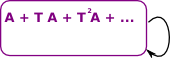Syntax
Axioms

## Example 2 - Natural Numbers

 If we start with the natural numbers and some endofunction on them, then we can generate the initial and terminal algebras.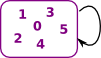Kleisli Eilenberg-Moore
initial terminal
Object

X = Zero() | Succ(X)

If we put in the usual notation for 'algebraic data types then we have to make:

• Zero=(1 -> N)
• Succ=(N -> N)

The algebraic type for natural numbers is:

N = 1+N

Although this notation is confusing in this case since, '1' represents zero and '+' represents disjoint union and not addition.

T AA
Arrow T(X) = least fixpoint of above
Zero+Succ(Zero())+Succ(Succ(Zero()))+...
A->AAxioms if X=Y
then succ(X)=succ(Y)
0+A = A + 0 = A
A+B=B+A
(A+B)+C=A+(B+C)
The functions zero and succ generate a carrier set, this has a structure in that it is ordered. Any algebra that obeys the axioms can represent the natural numbers.

## Example 3 - Integers

 Now extend the natural numbers example by adding negative numbers.Kleisli Eilenberg-Moore
initial terminal
Object A T AA
Arrow T A->ASyntax A=zero()
A=succ(A)
A=pre(A)
0()->T
+(T,T)->T
-T->T
Axioms

if A=B
then succ(A)=succ(B)
and pre(A)=pre(B)

pre(succ(A)) = A
succ(pre(A)) = A

0+A = A + 0 = A
A+(-A) = 0
A+B=B+A
(A+B)+C=A+(B+C)

The functions zero,succ and pre seem to generate the elements.

## Example 4 - Rational Numbers

 Now extend the natural numbers example by adding negative numbers.Kleisli Eilenberg-Moore
initial terminal
Object

X =

f(0) = 1

f(n+1) = ½ f(n)

T AA
Arrow T A->ASyntax A=zero()
A=succ(A)
A=pre(A)
0()->T
+(T,T)->T
-T->T
Axioms

if A=B
then succ(A)=succ(B)
and pre(A)=pre(B)

pre(succ(A)) = A
succ(pre(A)) = A

0+A = A + 0 = A
A+(-A) = 0
A+B=B+A
(A+B)+C=A+(B+C)

The functions zero,succ and pre seem to generate the elements.

## Example 5 - Algebra from Syntax

Can we create an algebraic theory from a (BNF) abstract syntax tree like this:

BNF Element (Example)

E ::= E | E '+' E | E '*' E | '-' E | N
N ::= (0..9)+

where:

• E is an expression in N.
• N is a number.How can we construct an expression? Can we form a monad?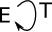So a unit for a monad could be:

N -> E N

Can this construct an expression using this? This does not appear to specify where in the heirarchy of the expression where to put this value.

Stack

it is only a tree if your base functor is a product functor. When you have a sum functor as the base functor it more closely resembles a stack machine - http://stackoverflow.com/questions/13352205/what-are-free-monads

 We could perhaps represent this with using multicategories like this: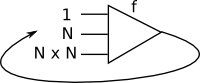## Example 6 - Words - Monad for Monoid

 On this page we discuss monoids in terms of a binary operation and an identity element, but there is another way to approach monoids (especially free monoids), that is as a singly linked list.We can convert between these notions of monoid using a 'fold' operation.The underlying category has objects which are either:

• characters
• lists of characters
• lists of lists of characters
• … and so on.

The endo-functor 'T' maps from this object to itself, in this case it maps:

• a character to a list of characters
• and a list of characters to a list of list of characters
• and so on.
 This endofunctor is equipped with two natural transformations: unit µ: 1 -> T multiplication (join) η: T×T -> T Where, µ removes the inner set of brackets - takes a list of lists and joins the inner lists ηT adds brackets to each of the inner elements T η adds brackets around the outside of an instance.So from this we can see the effect of various transformations:

 x -T-> (x) T wraps element in list x -ηx-> (x) η at x wraps element in list (x,y) -T-> ((x),(y)) T takes each element of list and wraps it in a sublist (x,y) -ηTx-> ((x,y)) η at T takes the whole list and wraps it in a sublist (x,y) -Tηx-> ((x),(y)) T•η at x takes each element of list and wraps it in a sublist ((x,y)) -µx-> (x,y) µ removes brackets - takes a list of lists and joins the inner lists (((x,y))) -µTx-> ((x,y)) -T-> -T-> -T->
 This satisfies the left and right triangle identities:and the associative square:

## Example 7 - Monad on Poset

An endofunctor on posets models closure. Posets don't have loops, therefore defined by fixpoints.

 Define T: P -> P with x <= T x T² x <= T x gives T² <= T (that is it is idempotent)Which gives an adjunction: ti

This is discussed as an adjunction on page here.

C List Graph
1 empty list
T list of elements free category on a graph
list of list of elements
unit (in algebra not monad) wrap in a list identity functor
multiplication (in algebra not monad) flatten (remove inner brackets) composition of two functors

### Computer Implementation of Algebra

Requires a carrier type to be defined then we need the algebra to be defined like this:

 Monoid `data MonF a = MEmpty | MAppend a a ` Ring `data RingF a = RZero | ROne | RNeg a | RAdd a a | Rmull a a `

To make this into an expression we need a recursive definition :

 Monoid `data MonExpr = MEmpty | MAppend Expr Expr ` Ring ```data RingExpr = RZero | ROne | RNeg RingExpr | RAdd RingExpr RingExpr | Rmull RingExpr RingExpr ```

Other sites:

This site:

Where I can, I have put links to Amazon for books that are relevant to the subject, click on the appropriate country flag to get more details of the book or to buy it from them.The Princeton Companion to Mathematics - This is a big book that attempts to give a wide overview of the whole of mathematics, inevitably there are many things missing, but it gives a good insight into the history, concepts, branches, theorems and wider perspective of mathematics. It is well written and, if you are interested in maths, this is the type of book where you can open a page at random and find something interesting to read. To some extent it can be used as a reference book, although it doesn't have tables of formula for trig functions and so on, but where it is most useful is when you want to read about various topics to find out which topics are interesting and relevant to you.